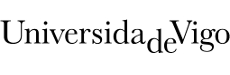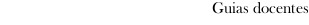Educational guide 2022_23Escola de Enxeñaría Industrial
 Grado en Ingeniería MecánicaSubjectsElasticity and additional topics in resistance of materialsContents
 Topic Sub-topic Fundamentals of elasticity Introduction to the theory of elasticity Stress analysis of elastic solids Strain Stress-strain relationships Two-dimensional elasticity Criteria of failure based in tensions Saint-Venants failure criterion Trescas failure criterion Von-Mises failure criterion Safety coefficient Bending Non uniform bending: Shear stresses. Zhuravski expression Principal stresses. Stress trajectories Bending and axial load: Normal stresses. Neutral axis Eccentric axial loads Kern of the cross-section Beams of different materials Bending. Statically indeterminate beams General method Settlements in fixed supports Continuous beams Simplifications in symmetric and antisymmetric beams Torsion Definition Coulombs fundamental theory Static torque diagrams Stress and angle of twist Statically indeterminate problems Combined loads Definition Bending and torsion loaded circular shafts Shear center Stress and strain calculation in plane-spatial structures Strain energy and energy methods Strain energy: Axial load/shearing loads/bending/torsion/general expression. Clapeyron's theorem Indirect and direct work MaxwellBetti Reciprocal Theorem Applications Castiglianos theorem. Mohr's integral. Applications Buckling Introduction Buckling and stability Eulers buckling. Critical load Buckling effective length Application limits of Eulers formula. Real buckling Eccentric compression of slim columns Shearing force and critical load
 Universidade de Vigo            | Reitoría | Campus Universitario | C.P. 36.310 Vigo (Pontevedra) | Spain | Tlf: +34 986 812 000